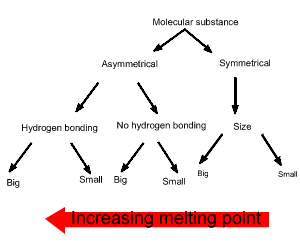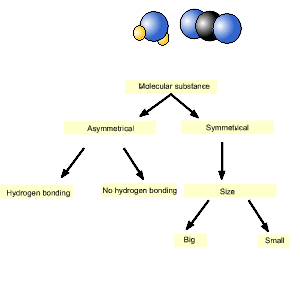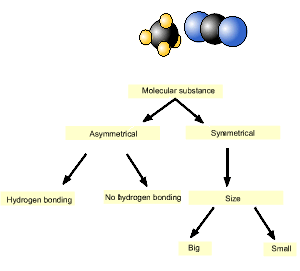Intermolecular forces Predicting melting temperature The intermolecular forces holding the molecules to each other in a molecular substance are generated by dispersion forces, dipole-dipole, and hydrogen bonding. The general order of increasing strength of the three types of intermolecular bonds is , dispersion forces, dipole-dipole and the strongest is hydrogen bonding. All molecules exhibit dispersion forces the strength of which depends on the size of the molecule. Hydrogen bonding is a strong form of dipole-dipole bonding. In predicting which molecular substance has the strongest inter-molecular bonding we have to see what forces are acting between its molecules. In order to predict the forces acting we need to know if the molecule: - is symmetrical; - has hydrogen bonding. Finally we need to know the number of electrons in the molecule in order to determine its relative size. Melting and boiling temperatures of substances are an indication of the relative strength of their intermolecular bonds, the stronger the intermolecular bonds the greater the melting temperature of the substance. Consider the simple diagram on the right. It is only used as a guide to sort molecules of the same size. As we follow the diagram downwards the substance furthest to the left has the highest melting temperature. Keep in mind this a simple method and overlap does occur between symmetrical molecules and asymmetrical molecules. A prime example is iodine (I2) which exists as a solid at room temperature due to its size which generates sizeable dispersion forces.Melting and boiling points are both indicators of the strength of inter-molecular bonding. Solid carbon dioxide and ice (water) are placed in a sealed vessel and heated. Which molecular substance has the highest melting point? Since water is the molecular substance on the left it has the highest melting point.Consider methane (CH4) and carbon dioxide (CO2). Which has the highest melting point? We will use the diagram, introduced above, to answer this question. As you can see from the animation on the right, carbon dioxide is on the left and hence has the highest melting point.1) Ethane, a symmetrical molecule, fluoromethane and methanol are three molecules of roughly the same size. Place them in order of increasing melting point. Solution 2) Carbon dioxide, methane and ethane are all symmetrical molecules. Place them in order of increasing melting point. 3) HCl and H2O both have 18 electrons. and were placed in a sealed vessel and cooled. Which compound solidified first? Explain using the diagram shown above. 4) a) Carbon tetrachloride (CCl4) has a boiling point of 76.8 oC. Trichloromethane (CHCl3) is a highly polar substance with strong inter-molecular attractions. Use the diagram above to reason which molecule has the highest boiling point. b) In fact trichloromethane has a boiling point of 61.1oC. What does this indicate about the importance of dispersion forces in inter-molecular attraction? 5) Describe the inter and intra molecular bonding of methane. Solution Continue.